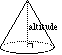index: click on a letter A B C D E F G H I J K L M N O P Q R S T U V W X Y Z A to Z index index: subject areas numbers & symbols sets, logic, proofs geometry algebra trigonometry advanced algebra & pre-calculus calculus advanced topics probability & statistics real world applications multimedia entrieswww.mathwords.com about mathwords website feedback

 Altitude of a Cone Height of a Cone The distance from the apex of a cone to the base. Formally, the shortest line segment between the apex of a cone and the (possibly extended) base. Altitude also refers to the length of this segment.See also Oblique cone, right cone, volume, surface area